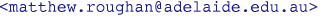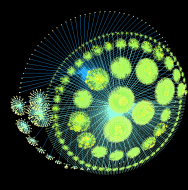# Complex-Network Modelling and Inference

 Home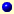NewsLecture NotesHandoutsAssignmentsTutorialsMatlab (protected) filesDataOther stuff
Lecture Notes
 Lecture 01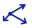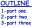Introduction and Course Summary Lecture 02Graph notation and representation Lecture 03Application: Bayesian Networks (and Complexity) Lecture 04Graph connectivity and traversal Lecture 05Nodes, paths and cycles Lecture 06Application: Genome Reconstruction Lecture 07Graph features Lecture 08Graph features (2) Lecture 09Application: PageRank Lecture 10Random Graphs: Erdos-Renyi random graphs Lecture 12Random Graphs: spatially-embedded and small-world networks Lecture 13Random Graphs: preferential-attachment models Lecture 14Random Graphs: HOT and COLD Lecture 15Modelling with Graphs, and Artificial Neural Networks Lecture 16Operations on graphs (unary operators) Lecture 17Operations on graphs (binary operators) Lecture 18Application: Graph Matching Lecture 19Shortest paths (Floyd-Warshall algorithm) Lecture 20Path algebras Lecture 21Path-problem algorithms Lecture 22Network Topology Measurement Lecture 23Network Sampling Lecture 24Network Tomography Lecture 25Network Topology Inference Lecture 26Revision

Matthew Roughan